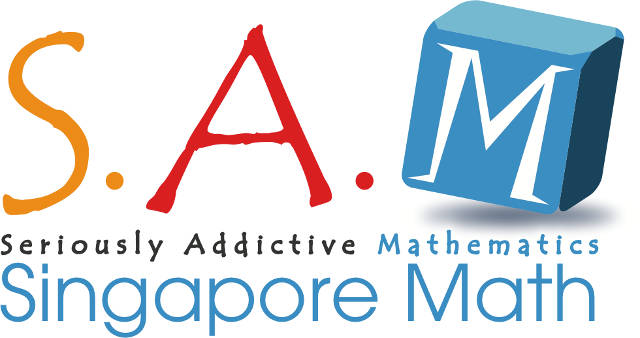What are the tools I can use to make maths interesting for children aged 4 – 6 years old?

The use of manipulates e.g. toy counters to teach number bonds. We call this the “concrete stage” of learning. This can be followed by drawing pictures or models to represent a problem to be solved e.g. 6 is ___ more than 2. We call this the “pictorial stage” of learning.How can I better guide children to solve math questions instead of just providing the solutions?

The following are steps to teach problem-solving:

1) Understand the problem i.e. what is being asked?

2) Choose the best method or heuristic like “Do I use guess-and-check, etc.

3) Working the method chosen i.e. the execution part

4) Looking back to assess the method used and decide if this is the best way forward for future problems.

Is it necessary for children to learn multiplication by heart?

When the concept of multiplication is taught well, learning by heart increases speed and accuracy. The basic concept of multiplication is adding the same number many times. E.g. 2 x 3 is 2 groups of 3 –> 3 + 3 = 6. Based on this a child can find 3 x 4 without learning by heart. Simply add 4 + 4 + 4 = 12.

Once the concept is understood, then memorising becomes useful. If a child does not understand the concept and forgets his tables, then he won’t be able to get the answer.

With double-digit addition and subtraction, how can I teach my child place values?

When introducing a new concept like place values, use manipulates like a place value chart. 1 is 1 one, 2 is 2 ones, 10 is 10 ones or 1 ten after regrouping etc. Show this using cubes or marbles. To add or subtract 2-digit numbers with 1-digit numbers, we may also teach them how to make 10 first, but this only comes after understanding number bonds. E.g. 12 – 3 = (10 + 2) – 3 –> 10 – 3 = 7 –> 7 + 2 = 9.

To understand better, watch this tutorial video: S.A.M Video Tutorial Series Part 5: Basic Math Concepts.

These questions were answered by Mr. Lau Chin Loong, Director and Co-founder of Seriously Addictive Mathematics.

This was first published in The New Age Parents Enrichment and Preschool Resource Guide.